# JP5485068B2 - Distributed amplifier - Google Patents

## Info

Publication number
JP5485068B2
JP5485068B2 JP2010173918A JP2010173918A JP5485068B2 JP 5485068 B2 JP5485068 B2 JP 5485068B2 JP 2010173918 A JP2010173918 A JP 2010173918A JP 2010173918 A JP2010173918 A JP 2010173918A JP 5485068 B2 JP5485068 B2 JP 5485068B2
Authority
JP
Japan
Prior art keywords
end
transmission line
output
connected
input
Prior art date
Legal status (The legal status is an assumption and is not a legal conclusion. Google has not performed a legal analysis and makes no representation as to the accuracy of the status listed.)
Active
Application number
JP2010173918A
Other languages
Japanese (ja)
Other versions
JP2012034291A (en
Inventor

Original Assignee

Priority date (The priority date is an assumption and is not a legal conclusion. Google has not performed a legal analysis and makes no representation as to the accuracy of the date listed.)
Filing date
Publication date
Application filed by 日本電信電話株式会社 filed Critical 日本電信電話株式会社
Priority to JP2010173918A priority Critical patent/JP5485068B2/en
Publication of JP2012034291A publication Critical patent/JP2012034291A/en
Application granted granted Critical
Publication of JP5485068B2 publication Critical patent/JP5485068B2/en
Application status is Active legal-status Critical
Anticipated expiration legal-status Critical

## Images

•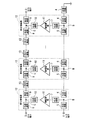•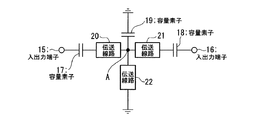••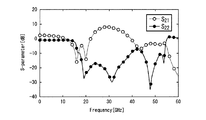•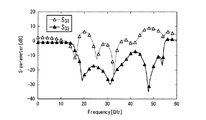•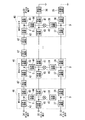•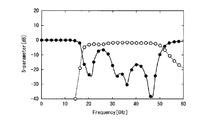•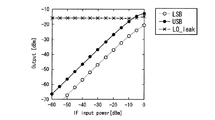•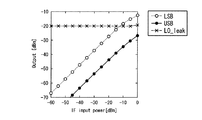•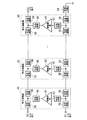••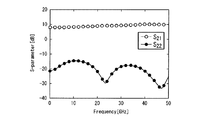•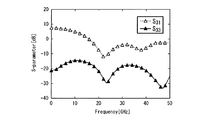••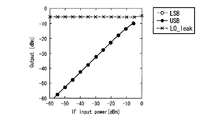•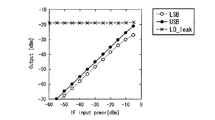## Description

The present invention relates to a distributed amplifier and a distributed mixer having a demultiplexing function for separating signals having different frequencies.

Conventionally, Non-Patent Document 1 reports a distributed circuit as an active circuit for amplifying and frequency-converting an input signal in a wide band.
The function of this distributed circuit will be described with reference to FIG. 10 using the distributed amplifier as an example. FIG. 10 is a diagram showing a circuit configuration of a conventional distributed amplifier.
In FIG. 10, reference numeral 51 is an input terminal, reference numerals 52 and 53 are output terminals, reference numeral 54 is a termination circuit, reference numerals 55, 56, 57, 58, 59 and 60 are transmission lines, reference numeral 61 is an amplifier unit, and reference numeral 62 is A unit amplifier is shown.

Next, FIG. 11 is a pseudo transmission line in each unit amplifier shown in FIG. 10 (a pseudo transmission line realizing 50Ω, and the capacitance between the input and ground of the transistors constituting the transmission lines 55 and 56 and the amplifier unit 61). (For example, when the amplifier unit 61 is a grounded emitter / source amplifier, an input pseudo transmission line composed of a base-emitter / gate-source capacitance), transmission lines 58 and 59, and outputs of transistors constituting the amplifier unit 61 FIG. 6 is a diagram illustrating a circuit configuration of a capacitance between the grounds (for example, an output pseudo transmission line including a collector-emitter / drain-source capacitance when the amplifier unit 61 is an emitter / source grounded amplifier).
In FIG. 11, reference numerals 71 and 72 are input / output terminals, reference numerals 73 and 74 are transmission lines, and reference numeral 75 is a capacitive element.
On the input side (input terminal 51 side) of the distributed amplifier of FIG. 10, transmission lines 55 and 56 in FIG. 10 correspond to the transmission lines 73 and 74 of FIG. 11, and the transistors constituting the amplifier unit 61 of FIG. The capacitance between the input and the ground corresponds to the capacitive element 75 in FIG.
On the other hand, on the output side (output terminal 53 side) of the distributed amplifier in FIG. 10, the transmission lines 58 and 59 in FIG. 10 correspond to the transmission lines 73 and 74 in FIG. 11, and constitute the amplifier unit 61 in FIG. The capacitance between the output and the ground of the transistor that corresponds to the capacitance element 75 corresponds.
In FIG. 11, the transmission lines 57 and 60 in FIG. 10 are omitted to simplify the description.

Here, if the inductance of the transmission lines 73 and 74 is L 0 , and the capacitance value of the capacitive element is C 0 , the transmission line is determined so that the characteristic impedance Z 0 of the pseudo transmission line is 50Ω, thereby cutting off the transmission line. It can be used as a 50Ω transmission line below the frequency fc.
Here, the characteristic impedance Z 0 and the cut-off frequency f c, respectively calculated by the following equation.
Z 0 = (L 0 / C 0 ) 1/2
f c = 1 / {π (L 0 / C 0 ) 1/2 }
By using the above-described pseudo transmission line as an input / output transmission line, it is possible to realize an amplifier (circuit) in which characteristic impedance is matched to 50Ω over a wide band.

Next, FIG. 12 shows an S parameter calculation result (forward transfer coefficient S) calculated in a configuration in which the input terminal 51 in FIG. 10 is the terminal 1, the output terminal 52 is the terminal 2, and the unit amplifiers 62 are connected in four stages. 21 and the reflection coefficient S 22 ) on the output side.
With the forward transmission coefficient S 21 in FIG. 12, the output signal is synthesized in the traveling direction (forward direction) in each unit amplifier, so that the gain from the output terminal 52 is about 8 dB over a wide band. You can see that it is obtained.

Further, FIG. 13 shows the calculation result of the S parameter (forward transmission coefficient S 31 and the forward transmission coefficient S 31 and the calculation result in the configuration in which the input terminal 51 in FIG. 10 is the terminal 1, the output terminal 53 is the terminal 3, and the unit amplifiers are connected in four stages. It is a figure which shows the reflection coefficient S33 ) of an output side.
The forward transfer coefficient S 31 in FIG. 13 prevents the output signal from being combined in the direction opposite to the traveling direction in each unit amplifier, so that the gain from the output terminal 53 is about 8 dB over a wide band. It turns out that it is not obtained.

Next, the operation of the distribution mixer will be described with reference to FIG. FIG. 14 is a diagram showing a circuit configuration of a conventional distributed mixer.
In FIG. 14, reference numeral 81 is an IF input terminal to which an IF (Intermediate Frequency) signal is input, reference numeral 82 is an LO input terminal to which an LO (Local Oscillation) signal is input, and reference numerals 83 and 84 are RF. (Radio Frequency; RF output terminal from which a signal is output), reference numerals 85 and 86 are termination circuits, reference numerals 87, 88, 89, 90, 91, 92 and 93 are transmission lines, reference numeral 95 is a mixer section, and reference numeral 96 is A unit mixer is shown. Each of the transmission lines 87, 88, 90, 91, 92, 93, and 94 realizes wideband characteristics by setting the line constants in the same manner as the input / output pseudo transmission lines of the distributed amplifier described above. be able to.

FIG. 15 shows a USB (Upper Side Band) side RF output signal output from the output terminal 83 of the distributed mixer and LSB (Lower Side Band) when six unit mixers in FIG. 14 are connected. It is a figure which shows the calculation result of the dependence with respect to the input power of IF signal of RF output signal of a lower sideband) side and LO leak output.
FIG. 16 shows a USB (Upper Side Band) side RF output signal output from an output terminal 84 of the distributed mixer and LSB (Lower Side Band) when six unit mixers in FIG. 14 are connected. It is a figure which shows the calculation result of the dependence with respect to the input power of IF signal of RF output signal of a lower sideband) side, and the leak output from LO signal input terminal.
15 and 16, the LO signal has a frequency of 25 GHz, and the IF signal has a frequency of 5 GHz. 15 and 16, it can be seen that an RF output signal (both USB side and LSB side) obtained by mixing the IF signal with the LO signal is output from the RF output terminal (83 or 84).

On the other hand, Non-Patent Document 2 discloses a right-handed / left-handed system having both the characteristics of a right-handed medium whose dielectric constant and permeability are both positive and a left-handed medium whose dielectric constant and permeability are both negative. A composite (CRLH: Composite Right / Left-Handed) line has been reported.
FIG. 2 is a diagram illustrating a configuration example of a unit cell of a CRLH line. In FIG. 2, reference numerals 15 and 16 are input / output terminals, reference numerals 17, 18 and 19 are capacitive elements, and reference numerals 20, 21 and 22 are transmission lines.
In the unit cell of the CRLH line, the transmission lines 20 and 21 and the capacitive element 18 are connected in series between the input / output terminals 15 and 16, and the connection point A of the transmission lines 20 and 21 is connected to the transmission line 22 and the capacitive element 19. Each is grounded in parallel.
That is, one end of the capacitive element 17 is connected to the input / output terminal 15. One end of the transmission line 20 is connected to the other end of the capacitive element 17. One end of the transmission line 21 is connected to the other end of the transmission line 20 at the connection point A. The capacitive element 18 has one end connected to the other end of the transmission line 21 and the other end connected to the input / output terminal 16. The capacitive element 19 has one end connected to the connection point A and the other end grounded. The transmission line 22 has one end connected to the connection point A and the other end grounded.

In the unit cell of the above CRLH line, the capacitance of the capacitor 17 and 18 and C 1, the capacitor 19 and C 2, the inductance of the transmission line 20 and 21 and L 1, the inductance of the transmission line 22 L 2 And Then, the capacitance values C 1 and C 2 and the inductances L 1 and L 2 are set so as to satisfy the following expression (1) indicating the angular frequency ω.
ω = 1 / (C 1 · L 1 ) 1/2 = 1 / (C 2 · L 2 ) 1/2 (1)
The input / output impedance Z is expressed by the following equation (2) for each frequency ω that satisfies the conditions of the above formula, and a band between the right-handed transmission band and the left-handed transmission band in the right-handed / left-handed composite line. The balance condition is such that the gap disappears, and the input / output impedance is constant.
Z = (L 2 / C 1 ) 1/2 = constant (2)

Next, FIG. 3 is a diagram showing an example of the calculation result of the transmission and reflection characteristics when the unit cells of the CRLH line shown in FIG. 2 are connected in three stages.
This FIG. 3 shows the capacitance on the GaAs substrate (dielectric constant 12.8, substrate thickness 100 μm) so that the above-described balance condition disappears between the band gap between the right-handed transmission band and the left-handed transmission band. The calculation result of the S parameter in which the values C 1 and C 2 and the inductances L 1 and L 2 are set and the unit cells of the CRLH line are connected in three stages is shown. Here, in the unit cell of the CRLH line in FIG. 2, the capacitive elements 17, 18 and 19 are 0.1 pF, the transmission lines 20 and 21 are configured with a line length of 370 μm and a line width of 5 μm. The transmission line 22 is a coplanar line having a line length of 235 μm and a line width of 5 μm, and the distance between the transmission line and the ground line is 37.5 μm. This CRLH line has an input / output impedance of 50Ω.

As can be seen from the calculation results of the forward transmission coefficient S 21 in FIG. 3, over a wide frequency of 50GHz from 18 GHz, it is understood that the 50Ω matching is achieved.
Also in the resonance frequency 25GHz determined by the inductance of the capacitance values and the transmission line of the capacitor, the reflection coefficient S 11 of the input side becomes less -7.5 dB, even 50Ω matching is achieved at the resonant frequency, right-handed / left It can be seen that there is no band gap between the right-handed transmission band and the left-handed transmission band in the system composite line.

I. D. Robertson and S.M. Lucyszyn, "RFIC and MMIC design and technology", pp. 213-225, The Institution of Electrical Engineers Atsushi Sanada, "Basics and Circuit Design of Right / Left-Handed Composite Metamaterials", MWE2007 Digest pp. 471-478, November 2008

As described above, FIG. 13 shows S parameter calculation results for the output terminal 53 when the input terminal 51 in FIG. 10 is the terminal 1, the output terminal 53 is the terminal 3, and unit amplifiers are connected in four stages.
However, the terminal position of the output terminal 53 is opposite to the traveling direction of the right-handed transmission path, and the output signal of each unit amplifier 62 is not synthesized as can be seen from FIG. For this reason, the output level of the signal output from the output terminal 53 is lower than the output level of the signal output from the output terminal 51 at the position in the traveling direction of the right-handed transmission path in FIG.
For this reason, the distributed amplifier having the configuration shown in FIG. 10 has a problem that the output terminal 53 cannot be effectively used as a terminal for outputting an output signal.

In the distributed mixer, as can be seen from the calculation results shown in FIGS. 15 and 16, the output levels of the RF output signals on the USB side and the LSB side of the mixing result output from the output terminals 83 and 84 in FIG. equal.
For this reason, when used in a transmitter, there is a problem in that it is impossible to make a setting for lowering the output level on either the USB side or the LSB side of the RF output signal.
Further, as apparent from the calculation results shown in FIGS. 15 and 16, there is a problem that the LO leakage output level is higher than the RF output signal.

In addition, since the CRLH line connects passive elements in multiple stages, there is a problem in that the output signal has a large passage loss.
When using two terminals, the signal input to the input / output terminal 15 in FIG. 2 is also output to the input / output terminal 16 in FIG. 2 even in the frequency band of the left-handed region. There is a problem that the circuit cannot be used separately from the signal.

The present invention has been made in view of such circumstances, and an amplifier capable of demultiplexing different frequency bands and a mixer capable of reducing LO leakage output level and capable of demultiplexing LSB and USB signals. The purpose is to provide.

The distributed amplifier according to the present invention is formed by connecting one end of a first transmission line, one end of a second transmission line, and one end of a third transmission line at a first connection point, in addition to the first transmission line. An input pseudo transmission line having a first input end and the other end of the second transmission line as a second input end, one end of a fourth transmission line, one end of a fifth transmission line, and a sixth One end of the transmission line is connected at a second connection point, the other end of the fourth transmission line is the first output end, and the other end of the fifth transmission line is the second output end. and the pseudo transmission line, an input terminal connected to the other end of said third transmission line, and the unit amplifier output terminal is composed of the other end is connected to the amplification portion of the sixth transmission line, the first One end of a seventh transmission line is connected to one end of the capacitive element, and the other end of the seventh transmission line and one end of the eighth transmission line are connected to a third connection point. One end of the second capacitive element is connected to the other end of the eighth transmission line, one end of the ninth transmission line is connected to the third connection point, and the ninth transmission line The other end of the third capacitor is grounded, one end of the third capacitor is connected to the third connection point, the other end of the third capacitor is grounded, and the other end of the first capacitor is connected to the first. And the other end of the second capacitive element is a second input / output terminal, which has a left-handed propagation characteristic in the first frequency band, and has a second frequency higher than the first frequency band. A distributed amplifier configured by connecting a plurality of left-hand / right-handed composite lines having right-handed propagation characteristics in a frequency band, and having a signal input terminal connected to the first input terminal of the unit amplifier in the first stage. The second input terminal is connected to the first input terminal of the next stage unit amplifier. A second input terminal of the unit amplifier at the final stage is connected to a termination circuit, a first signal output terminal is connected to the first output terminal of the unit amplifier at the first stage, and the second output terminal It said first input-output terminal of the left / right-handed composite line is connected, connected to the second input and output terminals of the left / right-handed composite line is to the first output terminal of the next stage of the unit amplifier The second output terminal of the unit amplifier in the final stage is connected to a second signal output terminal, and the left-hand / right-handed composite line has capacitances of the first and second capacitive elements, respectively. with a value of C 1, the capacitance value of said third capacitor and C 2, the inductance of the seventh and eighth transmission line and L 1, the inductance of the ninth transmission line and L 2, the following In order to satisfy the equation (1), the capacitance values C 1 and C 2 and N
Ductances L 1 and L 2 are set, and the upper limit of the first frequency band and the lower limit of the second frequency band are both frequencies corresponding to the angular frequency ω .
ω = 1 / (C 1 · L 1 ) 1/2 = 1 / (C 2 · L 2 ) 1/2 (1)

In the distributed amplifier according to the present invention, when an input signal in the first frequency band is input to the signal input terminal, an output signal is output from the first signal output terminal, and an input signal in the second frequency band Is input, an output signal is output from the second signal output terminal.

According to the present invention, in a distributed amplifier configured by connecting unit amplifiers in multiple stages, the frequency band of the right-handed region and the left-handed region of the left-handed region can be obtained by inserting the CRLH line between the connections of the output terminals of the unit amplifier. It becomes possible to control the transmission direction of the signal with the frequency band, and it is possible to demultiplex signals of different frequency bands and output them from different output terminals of the distributed amplifier.

Further, according to the present invention, in a distributed mixer configured by connecting unit mixers in multiple stages, the CRLH line is inserted between the connection of the RF output terminals of the unit mixer, so that the frequency band of the right-handed region and the left-handed region are It is possible to control the transmission direction of the signal with the frequency band of the system region, demultiplex the signals of the LSB and USB frequency bands, and output them from different output terminals of the distributed amplifier, and LO signal leakage The level can be kept low.

1 is a schematic block diagram illustrating a configuration example of a distributed amplifier according to a first embodiment of the present invention. It is a figure which shows the structural example of the CRLH line | wire in FIG. It is a figure which shows the calculation result of S parameter at the time of connecting the three CRLH line | wire of FIG. 2 in multistage in series. FIG. 2 is a diagram illustrating a calculation result of S parameters when four unit amplifiers are connected in series in the distributed amplifier of FIG. FIG. 2 is a diagram illustrating a calculation result of S parameters when four unit amplifiers are connected in series in the distributed amplifier of FIG. It is a schematic block diagram which shows the structural example of the distribution mixer by the 2nd Embodiment of this invention. It is a figure which shows the calculation result of S parameter at the time of connecting the three CRLH line | wire of FIG. 2 in multistage in series. It is a figure which shows the calculation result of the S parameter at the time of connecting the unit mixer in multiple stages in series in the distributed mixer of FIG. It is a figure which shows the calculation result of the S parameter at the time of connecting the unit mixer in multiple stages in series in the distributed mixer of FIG. It is a figure which shows the structure of the conventional distributed amplifier. It is a figure which shows the circuit structure of the pseudo transmission line which comprises each transmission line in FIG. It is a figure which shows the calculation result of the S parameter when four unit amplifiers are connected in multiple stages in series in the distributed amplifier of FIG. It is a figure which shows the calculation result of the S parameter when four unit amplifiers are connected in multiple stages in series in the distributed amplifier of FIG. It is a figure which shows the structure of the conventional distributed mixer. It is a figure which shows the calculation result of S parameter at the time of connecting six unit mixers in multistage in series in the distributed mixer of FIG. It is a figure which shows the calculation result of S parameter at the time of connecting six unit mixers in multistage in series in the distributed mixer of FIG.

<First Embodiment>
Hereinafter, a first embodiment of the present invention will be described with reference to the drawings. FIG. 1 is a schematic block diagram showing a configuration example of a distributed amplifier according to an embodiment of the present invention.
In the figure, the distributed amplifier is constructed by connecting unit amplifiers of reference numeral 12 in series in multiple stages, and a CRLH line of reference numeral 13 is inserted between the output terminals of each unit amplifier 12.
Reference numeral 1 is an input terminal of the distributed amplifier, and reference numerals 2 and 3 are output terminals of the distributed amplifier. Reference numeral 4 denotes a termination circuit. Here, the termination circuit 4 has an impedance set to 50Ω.
Therefore, in the distributed amplifier, the input signal input from the input terminal 1 is amplified in each unit amplifier, and an output signal (amplified signal) as an amplification result is output from the output terminal 2 or 3.

The unit amplifier 12 includes transmission lines denoted by reference numerals 5, 6, 7, 8, 9, and 10 and an amplifier section denoted by reference numeral 11 that amplifies an input signal and outputs it as an output signal.
In the unit amplifier 12, one end of the transmission line 5 (first input end of the unit amplifier 12) is connected to the input terminal 1, and the other end of the transmission line 5 and one end of the transmission line 6 are connected. The other end of the transmission line 6 is the second input end of the unit amplifier. The transmission line 7 has one end connected to the connection point B between the transmission line 5 and the transmission line 6 and the other end connected to the input end of the amplifier unit 11.

On the input side (input terminal 1 side) of the distributed amplifier in FIG. 1, the transmission lines 5 and 6 in the unit amplifier 12 in FIG. 1 correspond to the transmission lines 73 and 74 constituting the pseudo transmission line shown in FIG. The parasitic capacitance on the input side of the amplifier unit 11 in FIG. 1 corresponds to the capacitive element 75 in FIG.
On the other hand, on the output side (output terminal 3 side) of the distributed amplifier of FIG. 1, the transmission lines 8 and 9 in the unit amplifier 12 of FIG. 1 correspond to the transmission lines 73 and 74 constituting the pseudo transmission line shown in FIG. In addition, the parasitic capacitance on the output side of the amplifier unit 11 in FIG.
In FIG. 11, the transmission lines 7 and 10 in FIG. 1 are omitted for the sake of simplicity.

In the unit amplifier 12, one end of the transmission line 5 and one end of the transmission line 6 are connected at the connection point B, and one end of the transmission line 7 is connected to the connection point B. Here, the other end of the transmission line 5 is a first input end of the unit amplifier 12, and the other end of the transmission line 6 is a second input end of the unit amplifier 12.
In the unit amplifier 12, one end of the transmission line 8 and one end of the transmission line 9 are connected at the connection point C, and one end of the transmission line 10 is connected to the connection point C. Here, the other end of the transmission line 8 is a first output end of the unit amplifier 12, and the other end of the transmission line 9 is a second output end of the unit amplifier 12.
The amplifier unit 11 has an input terminal connected to the other end of the transmission line 7 and an output terminal connected to the other end of the transmission line 10.

As shown in FIG. 1, the distributed amplifier is configured by connecting a plurality of unit amplifiers 12 in series, that is, in multiple stages.
In the multistage connection of the unit amplifiers 12 in this distributed amplifier, the first stage unit amplifier 12 has a first input terminal connected to the input terminal 1, a first output terminal connected to the output terminal 3, and a second input terminal connected to the next stage. The unit amplifier 12 is connected to the first input terminal, and the second output terminal is connected to the first output terminal of the next stage unit amplifier 12 via the CRLH line 13.
In the multistage connection of the unit amplifiers 12, the final stage unit amplifier 12 has a first input terminal connected to the second input terminal of the previous unit amplifier 12 and a first output terminal connected to the previous stage via the CRLH line 13. The unit amplifier 12 is connected to the second output terminal, the second input terminal is terminated by the termination circuit 4, and the second output terminal is connected to the output terminal 2.

Further, in the multistage connection of the unit amplifiers 12, the unit amplifiers 12 between the first stage and the last stage have a first input terminal connected to the second input terminal of the preceding unit amplifier 12 and a first output terminal connected to the CRLH line 13 Is connected to the second output terminal of the unit amplifier 12 in the previous stage, the second input terminal is connected to the first input terminal of the unit amplifier 12 in the next stage, and the second output terminal is connected to the second output terminal via the CRLH line 13. It is connected to the first output terminal of the stage unit amplifier 12.
Therefore, in the distributed amplifier, between the input terminal 1 and the output terminal 3, and between the output terminal 2 and the termination circuit 4, the plurality of unit amplifiers 12 are connected to the second output terminal of the preceding stage unit amplifier and the second stage amplifier. A CRLH line 13 is inserted between one output end and a plurality of stages are connected in series.

Next, FIG. 2 is a diagram showing the configuration of the unit cell of the CRLH line as already described. FIG. 3 is a diagram illustrating an example of calculation results of transmission characteristics and reflection characteristics when the unit cells of the CRLH line illustrated in FIG. 2 are connected in three stages. The input / output impedances at the input / output terminal 15 and the input / output terminal 16 in FIG. 2 are set to 50Ω, similar to the characteristic impedance of the unit amplifier 12 described above. In FIG. 3, the horizontal axis indicates the frequency of the input signal, and the vertical axis indicates the calculation result (dB value) of the S parameter.
In FIG. 2, each of the capacitive elements 17, 18 and 19 is 0.1 pF, each of the transmission lines 20 and 21 is a line length of 37 μm × line width of 5 μm, and the distance between each line and the ground point is 37.5 μm. The transmission line 23 is a coplanar line having a line length of 235 μm × line width of 5 μm and a distance between the line and the ground point of 37.5 μm, and the input / output impedance is 50Ω as already described.

That is, the capacitance values C 1 and C 2 of the capacitive elements 17 and 18 and the inductances L 1 and L 2 of the transmission lines 20 and 21 are set so as to satisfy the above expression (1). . For this reason, the input / output impedance Z is expressed by the expression (2) with each frequency ω satisfying the condition of the expression (1), and in the right-handed / left-handed composite line, the right-handed transmission band and the left-handed transmission band are It becomes a balance condition in which the band gap disappears, and the input / output impedance becomes a constant value.

As shown in FIG. 3, in the frequency region of resonance frequency 25GHz in FIG 2, the reflection coefficient S 11 of the input side of the S parameter less and less -7.5 dB.
Therefore, in the CRLH line of FIG. 2, by setting the capacitance value and the inductance satisfying the expression (1), when this CRLH line is connected in three stages (three are connected in series), even at the resonance frequency, It can be seen that a reflection characteristic having a small reflection coefficient S11 can be obtained with respect to the input, impedance matching at 50Ω is achieved even at the resonance frequency, and no band gap in the frequency band is generated.

As shown in FIG. 1, the unit amplifiers 12 are connected in series in multiple stages, and the CRLH shown in FIG. 2 is provided between the second output terminal and the first output terminal of each of the preceding and succeeding unit amplifiers 12 in the pseudo transmission line. When the line 13 is inserted (inserted), as shown in FIG. 3, the CRLH line 13 is impedance-matched to 50Ω in the frequency band from 22 GHz to 50 GHz. For this reason, the pseudo transmission line of FIG. 1 to which the CRLH line 13 is added also maintains the matching condition of the CRLH line 13 and is impedance-matched to 50Ω in the frequency band from 22 GHz to 50 GHz.
Here, in the frequency band from 22 GHz to 25 GHz, the CRLH line 13 exhibits left-handed propagation characteristics, and an output signal (amplified signal) is output to the output terminal 3.
Further, in the frequency band from 25 GHz to 50 GHz, the CRLH line 13 exhibits a right-handed propagation characteristic, and an output signal is output to the output terminal 2.
That is, an output signal of a higher frequency band is output from the output terminal 2 as compared with the amplified output signal of the frequency band output from the output terminal 3.

Next, FIG. 4 is a simulation of the S parameter when the unit amplifier 12 is connected in four stages and the CRLH line 13 described in FIG. 2 is inserted in the pseudo transmission line in the distributed amplifier according to the present embodiment shown in FIG. It is a figure which shows the calculation result by. In FIG. 4, an input terminal 1 for definitive Figure 1 and terminal 1, an output terminal 2 and terminal 2, the S-parameters calculated in configuration of connecting the unit amplifier calculation result (in the forward transfer factor S 12 and the output The reflection coefficient S 22 ) is shown. In FIG. 4, the horizontal axis indicates the frequency of the input signal, and the vertical axis indicates the calculation result (dB value) of the S parameter.
In the S parameter, S 21 is a forward transmission coefficient, which indicates a propagation characteristic of a transmission wave propagated to the output terminal 2, and S 22 is an output-side reflection coefficient.

Next, FIG. 5 shows a simulation of S parameters when the unit amplifier 12 is connected in four stages and the CRLH line 13 described in FIG. 2 is inserted in the pseudo transmission line in the distributed amplifier according to the present embodiment shown in FIG. It is a figure which shows the calculation result by. In FIG. 5, the calculation result of the S parameter (forward transmission coefficient S 13 and the output side of the output side) calculated in the configuration in which the input terminal 1 in FIG. 1 is the terminal 1, the output terminal 3 is the terminal 3, and the unit amplifier is connected. The reflection coefficient S 33 ) is shown. In FIG. 5, the horizontal axis indicates the frequency of the input signal, and the vertical axis indicates the calculation result (dB value) of the S parameter.
In the S parameter, S 31 is a forward transmission coefficient, which indicates a propagation characteristic of a transmission wave propagated to the output terminal 3, and is a reflection coefficient on the output side of S 33 .

As can be seen from the characteristic of S 21 in FIG. 4 described above, the signal supplied from the input terminal 1 is amplified by the amplifier unit 11 because the CRLH line 13 exhibits a right-handed propagation characteristic in the frequency band of 25 GHz to 50 GHz. The signal is output from the output terminal 2.
Further, as can be seen from the characteristic of S 31 in FIG. 5, the signal supplied from the input terminal 1 is amplified by the amplifier unit 11 because the CRLH line 13 exhibits a left-handed propagation characteristic in the frequency band of 22 GHz to 25 GHz. The signal is output from the output terminal 3.

That is, the signal inputted from the terminal 1 is amplified by the unit amplifier, and the output terminal 2 is output in the frequency band showing the right-handed characteristic by the CRLH line 13 connected to the pseudo transmission line on the output side in the same manner as the normal distributed amplifier. (FIG. 4) and in the frequency band showing the left-handed characteristic, it is output to the output terminal 3 that is not output by a normal distributed amplifier (FIG. 5).
As described above, the distributed amplifier according to the present embodiment has a configuration in which unit amplifiers are connected in multiple stages. By inserting the CRLH line 13 between the output terminal connections in the pseudo transmission line of the unit amplifier, It is possible to control the transmission direction of the signal in the frequency band of the system region and the frequency band of the left-handed region, and demultiplex signals of different frequency bands and output them from different output terminals 2 and 3 of the distributed amplifier, respectively. Can do.

<Second Embodiment>
The second embodiment of the present invention will be described below with reference to the drawings. FIG. 6 is a schematic block diagram showing a configuration example of a distributed mixer according to an embodiment of the present invention.
In the figure, the distributed mixer is configured by connecting unit mixers 46 in series in multiple stages, and a CRLH line 47 is inserted between the output terminals of each unit mixer 46.
Reference numeral 31 denotes an IF input terminal of the distributed mixer, reference numeral 32 denotes an LO input terminal, and reference numerals 33 and 34 denote RF output terminals. Reference numerals 35 and 36 denote termination circuits. Here, the termination circuits 35 and 36 have an impedance set to 50Ω.
The distribution mixer mixes the LO signal input from the LO input terminal 32 and the IF signal input from the IF input terminal 31 in each unit mixer 46, and outputs the RF output from the RF output terminal 33 or 34 as a mixing result. Output a signal.

The unit mixer 46 has transmission lines 37, 38, 39, 40, 41, 42, 43, and 44, and a mixer section 45 that mixes IF signals and LO signals and outputs them as RF signals. ing.
In this unit mixer 46, one end of the transmission line 37 is connected to the IF input terminal 31, and the other end of the transmission line 37 and one end of the transmission line 38 are connected. The transmission line 39 has one end connected to a connection point D between the transmission line 37 and the transmission line 38, and the other end connected to the IF input end of the mixer unit 45.

On the IF signal input side (IF input terminal 31 side) of the distributed mixer of FIG. 6, the transmission lines 37 and 38 of the unit mixer 46 correspond to the transmission lines 73 and 74 constituting the pseudo transmission line shown in FIG. Further, the parasitic capacitance at the IF input end of the mixer unit 45 of FIG. 6 corresponds to the capacitive element 75 of FIG.
On the other hand, on the LO signal input side (LO input terminal 32 side) of the distributed mixer of FIG. 6, the transmission lines 40 and 41 of the unit mixer 46 in FIG. 6 are transmission lines 73 that constitute the pseudo transmission line shown in FIG. And 74, and the parasitic capacitance at the LO input end of the mixer unit 45 corresponds to the capacitive element 75.
Further, on the output side (RF output terminal 34 side) of the RF output signal of the distributed mixer of FIG. 6, the transmission lines 42 and 43 of the unit mixer 46 in FIG. 6 are the transmission lines constituting the pseudo transmission line shown in FIG. 73 and 74, and the parasitic capacitance at the RF output end of the mixer unit 45 corresponds to the capacitive element 75.
In FIG. 11, the transmission lines 39 and 44 in FIG. 6 are omitted for the sake of simplicity.

In the unit mixer 46, one end of the transmission line 37 and one end of the transmission line 38 are connected at the connection point D, and one end of the transmission line 39 is connected to the connection point D. Here, the other end of the transmission line 37 is a first LF input end of the unit mixer 46, and the other end of the transmission line 38 is a second LF input end of the unit mixer 46.
In the unit mixer 46, one end of the transmission line 40 and one end of the transmission line 41 are connected at a connection point E. Here, the other end of the transmission line 40 is a first LO input end of the unit mixer 46, and the other end of the transmission line 41 is a second LO input end of the unit mixer 46.
In the unit mixer 46, one end of the transmission line 42 and one end of the transmission line 43 are connected at the connection point F, and one end of the transmission line 44 is connected to the connection point F. Here, the other end of the transmission line 42 is a first RF output end of the unit mixer 46, and the other end of the transmission line 43 is a second RF output end of the unit mixer 46.
The mixer unit 45 has an IF terminal connected to the other end of the transmission line 39, an LO terminal connected to the connection point E, and an RF terminal connected to the other end of the transmission line 44.

As shown in FIG. 6, the distributed mixer is configured by connecting a plurality of unit mixers 46 in series, that is, in multiple stages.
In the multistage connection of the unit mixers 46 in this distributed mixer, the first stage unit mixer 46 has a first IF input terminal connected to the IF input terminal 31, a first LO input terminal connected to the LO input terminal 32, and a first RF output terminal connected to the first mixer input terminal 31. The second LO input terminal is connected to the RF output terminal 34, the second LO input terminal is connected to the first LO input terminal of the next stage unit mixer 46, and the second RF output terminal is connected to the first RF output terminal of the next stage unit mixer 46 via the CRLH line 47. It is connected to the.
In the multistage connection of the unit mixers 46, the final unit mixer 46 has a first IF input terminal connected to a second IF input terminal of the previous unit mixer 46, and a first LO input terminal connected to the second LO of the previous unit mixer 46. The first RF output terminal is connected to the second RF output terminal of the previous unit mixer 46 via the CRLH line 47, the second IF input terminal is terminated by the termination circuit 35, and the second LO input terminal is terminated. Termination processing is performed by the circuit 36, and the second RF output terminal is connected to the RF output terminal 33.

In the multistage connection of the unit mixers 46, the unit mixer 46 between the first stage and the last stage has a first IF input terminal connected to a second IF input terminal of the previous unit mixer 46, and a first LO input terminal connected to the previous unit. The first RF output terminal is connected to the second RF output terminal of the preceding unit mixer 46 via the CRLH line 47, and the second IF input terminal is connected to the first IF of the next unit mixer 46. The second LO input terminal is connected to the first LO input terminal of the next stage unit mixer 46, and the second RF output terminal is connected to the first RF output terminal of the next stage unit mixer 46 via the CRLH line 47. Has been.
Therefore, in the distributed mixer, a plurality of unit mixers 46 are connected between the IF input terminal 31, the LO input terminal 32, and the RF output terminal 34, and the termination circuit 35, the termination circuit 36, and the RF output terminal 33. A CRLH line 47 is interposed between the other end of the transmission line 43 of the unit mixer and one end of the transmission line 42 of the subsequent unit mixer, and a plurality of stages are connected in series.
Further, the CRLH line 47 in FIG. 6 has the configuration shown in FIG. 2, similarly to the CRLH line 13 in FIG. 1, and includes input / output terminals 15 and 16, capacitive elements 17, 18 and 19, transmission lines 20 and 21 And 22.

Next, FIG. 7 is a diagram illustrating an example of calculation results of transmission and reflection characteristics when the unit cells of the CRLH line illustrated in FIG. At this time, the input / output impedance of the CRLH line is set to 50Ω. In FIG. 7, the horizontal axis represents the frequency of the input signal, and the vertical axis represents the S parameter calculation result (dB value). In this calculation, each of the capacitive elements 17, 18 and 19 in FIG. 2 is 0.1 pF, each of the transmission lines 20 and 21 has a line length of 360 μm × line width of 5 μm, and the distance between each line and the ground point is 37. The transmission line 22 is a coplanar line having a line length of 240 μm × line width of 5 μm and a distance between the line and the ground point of 37.5 μm. The input / output impedance of the CRLH line 47 is set to 50Ω as described above.

Each parameter of the capacitance element and the inductance of the CRLH line 47 described above is set so that the frequency gap between the frequency band of the right-handed system characteristic and the frequency band of the left-handed system characteristic is widened as compared with the simulation in FIG. ing.
From the calculation result of FIG. 7, even in the resonance frequency 25 GHz band (band between 24 GHz and 26 GHz), the reflection coefficient S 11 in the S parameter is −6.5 dB, the reflection characteristic is lowered at the resonance frequency, and the passing loss is also reduced. It can be seen that the band gap is reduced to −3.5 dB.

As shown in FIG. 6, unit mixers 46 are connected in multiple stages, for example, six stages in series, and the other end of each transmission line 43 in each of the preceding unit mixers 47 and the transmission line in each of the subsequent unit mixers 46 in the pseudo transmission line. 2, when the CRLH line 47 shown in FIG. 2 is inserted (inserted), as shown in FIG. 6, the CRLH line 47 has a frequency band of 22 GHz to 24 GHz and 26 GHz to 50 GHz, respectively. Impedance matched to 50Ω. Therefore, the pseudo transmission line of FIG. 6 to which the CRLH line 47 is added also maintains the matching condition of the CRLH line 47 and is impedance-matched to 50Ω in the frequency band from 22 GHz to 24 GHz and from 26 GHz to 50 GHz. That is, at 25 GHz, the impedance is not 50Ω.

Here, in the frequency band from 22 GHz to 24 GHz (LSB on the low frequency band side), the CRLH line 47 exhibits left-handed propagation characteristics, and an RF output signal is output to the RF output terminal 34.
In the frequency band from 26 GHz to 50 GHz (high frequency band side USB), the CRLH line 47 exhibits right-handed propagation characteristics, and an RF output signal is output to the RF output terminal 33.
Compared with the frequency output from the RF output terminal 34 in two frequency bands sandwiching the 25 GHz frequency, an RF output signal having a higher frequency is output from the RF output terminal 33.

Next, FIG. 8 shows an RF output signal when the CRLH line 47 described in FIG. 2 is inserted into the pseudo transmission line in the distributed mixer according to the present embodiment shown in FIG. It is a figure which shows the calculation result by the simulation of the dependence of IF input of LO leak output. In FIG. 8, the horizontal axis indicates the level (dB value) of the input IF signal, and the vertical axis indicates the calculation result (dB value) of the output RF signal.
FIG. 8 shows the dependency of the RF output (USB and LSB) output from the RF output terminal 33 in FIG. 6 and the LO leakage output LO_leak on the IF input signal. In this simulation, the frequency of the LO signal is 25 GHz where the reflection characteristics and the passage loss are reduced, and the frequency of the IF signal is 5 GHz.
From FIG. 8, it can be seen that by setting the frequency of the LO signal to 25 GHz, which is the band gap of the CRLH line 47, the signal level of LO_leak is reduced by about 10 dB compared to the conventional example of FIG.
Further, it can be seen from FIG. 8 that the LSB signal level is 10 dB lower than that of the USB, which is the desired signal, which is a higher frequency band than the LSB.

Next, FIG. 9 shows an RF output signal when the unit mixer 46 is connected in six stages in the distributed mixer according to the present embodiment shown in FIG. 6 and the CRLH line 47 described in FIG. It is a figure which shows the calculation result by the simulation of the dependence of IF input of LO leak output. In FIG. 9, the horizontal axis indicates the level (dB value) of the input IF signal, and the vertical axis indicates the calculation result (dB value) of the output RF signal.
FIG. 9 shows the dependency of the RF output (USB and LSB) output from the RF output terminal 34 and the LO leakage output LO_leak on the IF input signal in FIG. In this simulation, the frequency of the LO signal is 25 GHz where the reflection characteristics and the passage loss are reduced, and the frequency of the IF signal is 5 GHz.
From FIG. 9, it can be seen that by setting the frequency of the LO signal to 25 GHz, which is the band gap of the CRLH line 47, the level of the LO_leak signal is lowered compared to the conventional example of FIG.
Further, it can be seen from FIG. 9 that the USB signal level is suppressed by 15 dB or more compared to the LSB which is the desired signal.

As described above, the distributed mixer of the present embodiment has a configuration in which the unit mixers 46 are connected in multiple stages, and the CRLH line 47 is inserted between the RF output terminal connections in the pseudo transmission line of the unit mixer 46. Thus, it becomes possible to control the transmission direction of the USB signal set in the frequency band of the right-handed region and the LSB signal set in the frequency band of the left-handed region, demultiplexing signals in different frequency bands, and LO The leakage output level can be reduced and output from different RF output terminals 33 and 34 of the distributed mixer, respectively.
In the distributed mixer of this embodiment, the frequency of the LO signal is set in the frequency band of the left-handed system region, and the frequency of the USB signal is set in the frequency band of the right-handed system region, so that the USB set in the frequency band of the right-handed region. The transmission direction of the LO signal set in the frequency band of the signal and the left-handed system region can be controlled, the LO leakage output level can be reduced, and the USB signal can be output from the RF output terminal 33.
Similarly, in the distributed mixer of the present embodiment, the frequency of the LO signal is set in the frequency band of the right-handed system region, and the frequency of the LSB signal is set in the frequency band of the left-handed system region. The transmission direction of the set LSB signal and the LO signal set in the right-handed region can be controlled, the LO leakage output level can be reduced, and the LSB signal can be output from the RF output terminal 34.

The embodiment of the present invention has been described in detail with reference to the drawings. However, the specific configuration is not limited to this embodiment, and includes design and the like within a scope not departing from the gist of the present invention.
In other words, all of the above-described embodiments are illustrative of the embodiments of the present invention and are not intended to be limiting, and the present invention can be implemented in various modifications.
Therefore, the scope of the present invention is defined only by the claims and their equivalents.

DESCRIPTION OF SYMBOLS 1 ... Input terminal 2, 3, 33, 34 ... Output terminal 4, 35, 36 ... Termination circuit 5, 6, 7, 8, 9, 10, 20, 21, 22 ... Transmission line 11 ... Amplifier part 12 ... Unit amplifier DESCRIPTION OF SYMBOLS 13, 47 ... CRLH line 15, 16 ... Input / output terminal 17, 18, 19 ... Capacitance element 31 ... IF input terminal 32 ... LO input terminal 37, 38, 39, 40, 41, 42, 43, 44 ... Transmission line 45 ... Mixer part 46 ... Unit mixer

## Claims (2)

1. One end of the first transmission line, one end of the second transmission line, and one end of the third transmission line are connected at a first connection point, and the other end of the first transmission line is connected to the first input terminal. An input pseudo transmission line having the other end of the second transmission line as the second input end, one end of the fourth transmission line, one end of the fifth transmission line, and one end of the sixth transmission line An output pseudo transmission line having the other end of the fourth transmission line as a first output end and the other end of the fifth transmission line as a second output end; A unit amplifier including an amplifying unit having an input terminal connected to the other end of the third transmission line and an output terminal connected to the other end of the sixth transmission line;
One end of a seventh transmission line is connected to one end of the first capacitive element, the other end of the seventh transmission line and one end of the eighth transmission line are connected at a third connection point, and a second capacitor One end of the element is connected to the other end of the eighth transmission line, one end of the ninth transmission line is connected to the third connection point, the other end of the ninth transmission line is grounded, One end of the capacitive element is connected to the third connection point, the other end of the third capacitive element is grounded, the other end of the first capacitive element is used as a first input / output terminal, and the second The other end of the capacitor element is the second input / output terminal, and has a left-handed propagation characteristic in the first frequency band, and a right-handed propagation characteristic in the second frequency band having a frequency higher than the first frequency band. A left / right handed composite track,
Is a distributed amplifier configured by connecting a plurality of
A signal input terminal is connected to the first input terminal of the unit amplifier at the first stage, the second input terminal is connected to the first input terminal of the unit amplifier at the next stage, and the unit amplifier at the final stage. The second input end of the
A first signal output terminal connected to the first output of the unit amplifier of the first stage, the first output terminal and said second output terminal the left / right-handed composite line is connected, the left / the second input and output terminals of the right-handed composite line is connected to the first output terminal of the next stage of the unit amplifier, the second output of the unit amplifier of the last stage of the second Connected to the signal output terminal ,
The left-hand / right-handed composite lines are respectively
The capacitance value of the first and second capacitive elements and C 1, the capacitance value of said third capacitor and C 2, the inductance of the seventh and eighth transmission line and L 1, the ninth The capacitance values C 1 and C 2 and the inductances L 1 and L 2 are set so that the inductance of the transmission line is L 2, and the following expression (1) is satisfied, and the upper limit of the first frequency band and A distributed amplifier characterized in that all of the lower limits of the second frequency band are frequencies corresponding to the angular frequency ω .
ω = 1 / (C 1 · L 1 ) 1/2 = 1 / (C 2 · L 2 ) 1/2 (1)
2. When an input signal of the first frequency band is input to the signal input terminal, an output signal is output from the first signal output terminal, and when an input signal of the second frequency band is input, The distributed amplifier according to claim 1, wherein an output signal is output from the second signal output terminal.
JP2010173918A 2010-08-02 2010-08-02 Distributed amplifier Active JP5485068B2 (en)

## Priority Applications (1)

Application Number Priority Date Filing Date Title
JP2010173918A JP5485068B2 (en) 2010-08-02 2010-08-02 Distributed amplifier

## Applications Claiming Priority (1)

Application Number Priority Date Filing Date Title
JP2010173918A JP5485068B2 (en) 2010-08-02 2010-08-02 Distributed amplifier

## Publications (2)

Publication Number Publication Date
JP2012034291A JP2012034291A (en) 2012-02-16
JP5485068B2 true JP5485068B2 (en) 2014-05-07

# Family

## Family Applications (1)

Application Number Title Priority Date Filing Date
JP2010173918A Active JP5485068B2 (en) 2010-08-02 2010-08-02 Distributed amplifier

## Country Status (1)

JP (1) JP5485068B2 (en)

## Families Citing this family (3)

* Cited by examiner, † Cited by third party
Publication number Priority date Publication date Assignee Title
JP5689841B2 (en) * 2012-02-28 2015-03-25 日本電信電話株式会社 Directional coupled mixer circuit
JP6124299B2 (en) * 2013-08-26 2017-05-10 日本電信電話株式会社 Distributed mixer
JP6290706B2 (en) * 2014-05-14 2018-03-07 日本電信電話株式会社 Combiner and mixer

## Family Cites Families (3)

* Cited by examiner, † Cited by third party
Publication number Priority date Publication date Assignee Title
US4662000A (en) * 1985-04-15 1987-04-28 Raytheon Company Frequency conversion circuits
JP3865043B2 (en) * 2001-04-06 2007-01-10 日本電気株式会社 Return loss suppression circuit
US8026854B2 (en) * 2006-07-14 2011-09-27 Yamaguchi University Stripline-type composite right/left-handed transmission line or left-handed transmission line, and antenna that uses same

## Also Published As

Publication number Publication date
JP2012034291A (en) 2012-02-16

## Similar Documents

Publication Publication Date Title
US8289102B2 (en) Directional coupler
JP5169844B2 (en) Directional coupler
JP2010062614A (en) Voltage controlled oscillator, mmic, and high frequency radio apparatus
CN102640350A (en) Metamaterial power amplifier systems
US20110210786A1 (en) Doherty Amplifier with Input Network Optimized for MMIC
US7440729B2 (en) Apparatus, methods and articles of manufacture for output impedance matching using multi-band signal processing
US20100001802A1 (en) Integrated doherty type amplifier arrangement with high power efficiency
US7750756B2 (en) Matching circuit
US20160268994A1 (en) Reconfigurable directional coupler with a variable coupling factor
US8704575B2 (en) Tunable active directional couplers
US20130099866A1 (en) System and Method for a Multi-Band Power-Amplifier
US7245186B2 (en) Bandpass amplifier
JP3865043B2 (en) Return loss suppression circuit
KR101156450B1 (en) Multiband matching circuit and multiband power amplifier
US9209511B2 (en) Doherty power amplifier network
TWI496413B (en) Distributed amplifier with improved stabilization
US7521995B1 (en) Inverted doherty amplifier with increased off-state impedence
US7187231B2 (en) Apparatus, methods and articles of manufacture for multiband signal processing
EP2312746B1 (en) Final three-way power synthesizing amplifier circuit for mobile communication base station system power amplifier
CN102142812B (en) Doherty power amplifier
US9647611B1 (en) Reconfigurable power splitters and amplifiers, and corresponding methods
KR20120018082A (en) Multiband matching circuit
JP4867346B2 (en) High frequency amplifier
CN1391354A (en) Efficient high-frequency amplifier and radio transmitter

## Legal Events

Date Code Title Description
A621 Written request for application examination

Free format text: JAPANESE INTERMEDIATE CODE: A621

Effective date: 20130129

A711 Notification of change in applicant

Free format text: JAPANESE INTERMEDIATE CODE: A711

Effective date: 20130129

A521 Written amendment

Free format text: JAPANESE INTERMEDIATE CODE: A821

Effective date: 20130129

RD02 Notification of acceptance of power of attorney

Effective date: 20130605

Free format text: JAPANESE INTERMEDIATE CODE: A7422

RD04 Notification of resignation of power of attorney

Effective date: 20130725

Free format text: JAPANESE INTERMEDIATE CODE: A7424

A977 Report on retrieval

Free format text: JAPANESE INTERMEDIATE CODE: A971007

Effective date: 20130821

A131 Notification of reasons for refusal

Effective date: 20130903

Free format text: JAPANESE INTERMEDIATE CODE: A131

A521 Written amendment

Free format text: JAPANESE INTERMEDIATE CODE: A523

Effective date: 20131105

TRDD Decision of grant or rejection written
A01 Written decision to grant a patent or to grant a registration (utility model)

Free format text: JAPANESE INTERMEDIATE CODE: A01

Effective date: 20140212

A61 First payment of annual fees (during grant procedure)

Effective date: 20140219

Free format text: JAPANESE INTERMEDIATE CODE: A61

R150 Certificate of patent (=grant) or registration of utility model

Ref document number: 5485068

Free format text: JAPANESE INTERMEDIATE CODE: R150

Country of ref document: JP